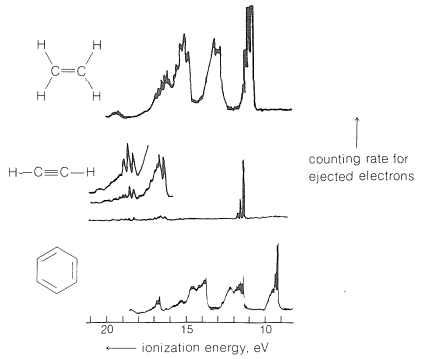# 27.6: Photoelectron Spectroscopy

$$\newcommand{\vecs}{\overset { \rightharpoonup} {\mathbf{#1}} }$$ $$\newcommand{\vecd}{\overset{-\!-\!\rightharpoonup}{\vphantom{a}\smash {#1}}}$$$$\newcommand{\id}{\mathrm{id}}$$ $$\newcommand{\Span}{\mathrm{span}}$$ $$\newcommand{\kernel}{\mathrm{null}\,}$$ $$\newcommand{\range}{\mathrm{range}\,}$$ $$\newcommand{\RealPart}{\mathrm{Re}}$$ $$\newcommand{\ImaginaryPart}{\mathrm{Im}}$$ $$\newcommand{\Argument}{\mathrm{Arg}}$$ $$\newcommand{\norm}{\| #1 \|}$$ $$\newcommand{\inner}{\langle #1, #2 \rangle}$$ $$\newcommand{\Span}{\mathrm{span}}$$ $$\newcommand{\id}{\mathrm{id}}$$ $$\newcommand{\Span}{\mathrm{span}}$$ $$\newcommand{\kernel}{\mathrm{null}\,}$$ $$\newcommand{\range}{\mathrm{range}\,}$$ $$\newcommand{\RealPart}{\mathrm{Re}}$$ $$\newcommand{\ImaginaryPart}{\mathrm{Im}}$$ $$\newcommand{\Argument}{\mathrm{Arg}}$$ $$\newcommand{\norm}{\| #1 \|}$$ $$\newcommand{\inner}{\langle #1, #2 \rangle}$$ $$\newcommand{\Span}{\mathrm{span}}$$$$\newcommand{\AA}{\unicode[.8,0]{x212B}}$$

The excitation of electrons to higher energy states through absorption of visible and ultraviolet light (usually covering the range of wavelengths from $$200 \: \text{nm}$$ to $$780 \: \text{nm}$$) is discussed in Sections 9-9 and 28-1. We now will consider what happens on absorption of much shorter wavelength, more energetic, photons.

When radiation of wavelengths on the order of $$120 \: \text{nm}$$ is absorbed by a molecule of ethene, the excited state has just sufficient energy (about $$250 \: \text{kcal mol}^{-1}$$) to cause the most loosely bound electron to be ejected. With radiation of still shorter wavelength, such as the $$58.4 \: \text{nm}$$ $$\left( 490 \: \text{kcal mol}^{-1} \right)$$ provided by a helium discharge tube, these electrons will have, by the Einstein law, a kinetic energy of $$\left( 490 - 250 \right) = 240 \: \text{kcal mol}^{-1}$$. More tightly bound electrons also can be ejected by $$58.4 \: \text{nm}$$ radiation, and they will have kinetic energies $$E = h \nu - I$$, in which $$h \nu$$ is the energy of the absorbed radiation $$\left( 490 \: \text{kcal mol}^{-1} \right)$$ and $$I$$ is the ionization energy. If we know $$h \nu$$ and measure the number of ejected electrons as a function of their kinetic energies, we can derive a spectrum that shows how the probability of excitation correlates with the ionization energy. Such spectra, called photoelectron spectra, are shown in Figure 27-10 for gaseous ethene, ethyne, and benzene and are quite individualistic. Considerable fine structure is observed as the consequence of a considerable spread in the vibrational levels of the excited state.Figure 27-10: Photoelectron spectra of ethene, ethyne, and benzene induced by $$58.4$$-$$\text{nm}$$ radiation from a helium-discharge lamp. For ethyne, the left part of the spectrum is shown with three different sensitivity levels. The horizontal scale here is in units of electron volts $$\left( \text{eV} \right)$$, which can be converted to $$\text{kcal mol}^{-1}$$ by multiplying by 23.06. (Published by permission of A. D. Baker and D. W. Turner, and of Accounts of Chemical Research.)

Substitution and conjugation have substantial effects on ionization energies. We have mentioned how methyl groups are able by their electron-donating power to stabilize carbon cations more than hydrogens do (Section 8-7B). The same effect is very prominent in the ionization of alkenes, the lowest energy required to eject an electron from 1-butene being about $$11 \: \text{kcal mol}^{-1}$$ greater than from cis- or trans-2-butene. The corresponding differences for 1-hexene and 2,3-dimethyl-2-butene are about $$27 \: \text{kcal mol}^{-1}$$. Conjugation produces similar effects. The lowest energy required to eject an electron from 1,4-pentadiene with isolated double bonds is $$21 \: \text{kcal mol}^{-1}$$ greater than for the isomeric 1,3-pentadiene with conjugated double bonds.

Photoelectron spectroscopy also can be carried on with x rays as the source of excitation and in this form often is called "ESCA" (Electron Spectroscopy for Chemical Analysis). The x rays used have wavelengths on the order of $$0.9 \: \text{nm}$$ $$\left( 32,000 \: \text{kcal mol}^{-1} \right)$$ and the energies involved are more than ample to cause ejection of electrons form inner shells as well as from valence shells. An x-ray photoelectron spectrum of the carbon $$1s$$ electrons of ethyl trifluoroethanoate is shown in Figure 27-11. This spectrum is extremely significant in that it shows four different peaks - one for each chemically different carbon present. What this means is that the energy required to eject a $$1s$$ electron of carbon depends on the chemical state of the carbon. The energy range for this compound is fully $$185 \: \text{kcal mol}^{-1}$$ of the approximately $$6700 \: \text{kcal mol}^{-1}$$ required to eject a $$1s$$ electron. This form of spectroscopy is especially well suited to the study of solid surfaces and is being used widely for the characterization of solid catalysts.Figure 27-11: Carbon $$1s$$ x-ray photoelectron spectrum of ethyl trifluoroethanoate. The zero point is $$291.2 \: \text{eV}$$. (Kindly supplied by Professor K. Siegbahn.)